# fillmissing

Fill missing values

## Syntax

``F = fillmissing(A,'constant',v)``
``F = fillmissing(A,method)``
``F = fillmissing(A,movmethod,window)``
``F = fillmissing(A,fillfun,gapwindow)``
``F = fillmissing(___,dim)``
``F = fillmissing(___,Name,Value)``
``````[F,TF] = fillmissing(___)``````

## Description

example

````F = fillmissing(A,'constant',v)` fills missing entries of an array or table with the constant value `v`. If `A` is a matrix or multidimensional array, then `v` can be either a scalar or a vector. When `v` is a vector, each element specifies the fill value in the corresponding column of `A`. If `A` is a table or timetable, then `v` can also be a cell array whose elements contain fill values for each table variable.Missing values are defined according to the data type of `A`: `NaN` — `double`, `single`, `duration`, and `calendarDuration``NaT` — `datetime``<missing>` — `string``<undefined>` — `categorical``' '` — `char``{''}` — `cell` of character arrays If `A` is a table, then the data type of each column defines the missing value for that column.```

example

````F = fillmissing(A,method)` fills missing entries using the method specified by `method`. For example, `fillmissing(A,'previous')` fills missing entries with the previous non-missing entry of `A`.```

example

````F = fillmissing(A,movmethod,window)` fills missing entries using a moving window mean or median with window length `window`. For example, `fillmissing(A,'movmean',5)` fills data with a moving average using a window length of 5.```

example

````F = fillmissing(A,fillfun,gapwindow)` fills gaps of missing entries using a custom method specified by a function handle `fillfun` and a fixed window surrounding each gap from which the fill values are computed. `fillfun` must have the input arguments `xs`, `ts`, and `tq`, which are vectors containing the sample data `xs` of length `gapwindow`, the sample data locations `ts` of length `gapwindow`, and the missing data locations `tq`. The locations in `ts` and `tq` are a subset of the sample points vector.```

example

````F = fillmissing(___,dim)` specifies the dimension of `A` to operate along. By default, `fillmissing` operates along the first dimension whose size does not equal 1. For example, if `A` is a matrix, then `fillmissing(A,2)` operates across the columns of `A`, filling missing data row by row.```

example

````F = fillmissing(___,Name,Value)` specifies additional parameters for filling missing values using one or more name-value pair arguments. For example, if `t` is a vector of time values, then `fillmissing(A,'linear','SamplePoints',t)` interpolates the data in `A` relative to the times in `t`.```

example

``````[F,TF] = fillmissing(___)``` also returns a logical array corresponding to the entries of `A` that were filled.```

## Examples

collapse all

Create a vector that contains `NaN` values and replace each `NaN` with the previous non-missing value.

```A = [1 3 NaN 4 NaN NaN 5]; F = fillmissing(A,'previous')```
```F = 1×7 1 3 3 4 4 4 5 ```

Create a 2-by-2 matrix with a `NaN` value in each column. Fill `NaN` with `100` in the first column and `1000` in the second column.

`A = [1 NaN; NaN 2]`
```A = 2×2 1 NaN NaN 2 ```
`F = fillmissing(A,'constant',[100 1000])`
```F = 2×2 1 1000 100 2 ```

Use interpolation to replace `NaN` values in non-uniformly sampled data.

Define a vector of non-uniform sample points and evaluate the sine function over the points.

```x = [-4*pi:0.1:0, 0.1:0.2:4*pi]; A = sin(x);```

Inject `NaN` values into `A`.

`A(A < 0.75 & A > 0.5) = NaN;`

Fill the missing data using linear interpolation, and return the filled vector `F` and the logical vector `TF`. The value 1 (`true`) in entries of `TF` corresponds to the values of `F` that were filled.

`[F,TF] = fillmissing(A,'linear','SamplePoints',x);`

Plot the original data and filled data.

```plot(x,A,'.', x(TF),F(TF),'o') xlabel('x'); ylabel('sin(x)') legend('Original Data','Filled Missing Data')```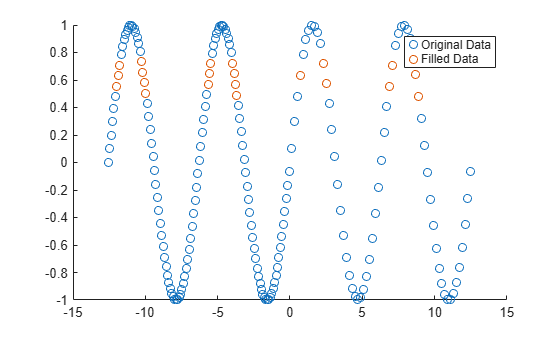Use a moving median to fill missing numeric data.

Create a vector of sample points `x` and a vector of data `A` that contains missing values.

```x = linspace(0,10,200); A = sin(x) + 0.5*(rand(size(x))-0.5); A([1:10 randi([1 length(x)],1,50)]) = NaN; ```

Replace `NaN` values in `A` using a moving median with a window of length 10, and plot both the original data and the filled data.

```F = fillmissing(A,'movmedian',10); plot(x,F,'r.-',x,A,'b.-') legend('Filled Missing Data','Original Data')```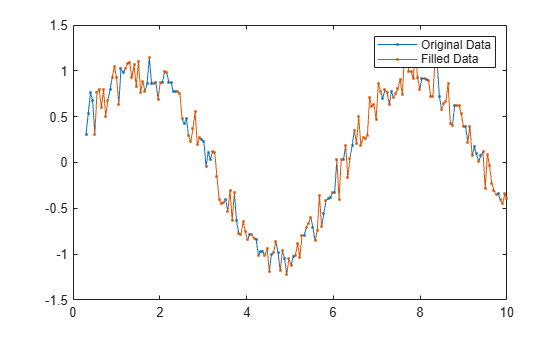Define a custom function to fill `NaN` values with the previous nonmissing value.

Define a vector of sample points `t` and a vector of corresponding data `A` containing `NaN` values. Plot the data.

```t = 10:10:100; A = [0.1 0.2 0.3 NaN NaN 0.6 0.7 NaN 0.9 1]; plot(t,A,'o')```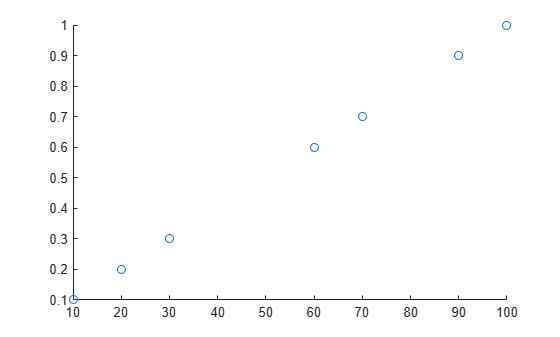Use the local function `forwardfill` (defined at the end of the example) to fill missing gaps with the previous nonmissing value. The function handle inputs include:

• `xs` — data values used for filling

• `ts` — locations of the values used for filling relative to the sample points

• `tq` — locations of the missing values relative to the sample points

• `n` — number of values in the gap to fill

```n = 2; gapwindow = [10 0]; [F,TF] = fillmissing(A,@(xs,ts,tq) forwardfill(xs,ts,tq,n),gapwindow,'SamplePoints',t);```

The gap window value `[10 0]` tells `fillmissing` to consider one data point before a missing gap and no data points after a gap, since the previous nonmissing value is located 10 units prior to the gap. The function handle input values determined by `fillmissing` for the first gap are:

• `xs = 0.3`

• `ts = 30`

• `tq = [40 50]`

The function handle input values for the second gap are:

• `xs = 0.7`

• `ts = 70`

• `tq = 80`

Plot the original data and the filled data.

`plot(t,A,'o',t(TF),F(TF),'ro')`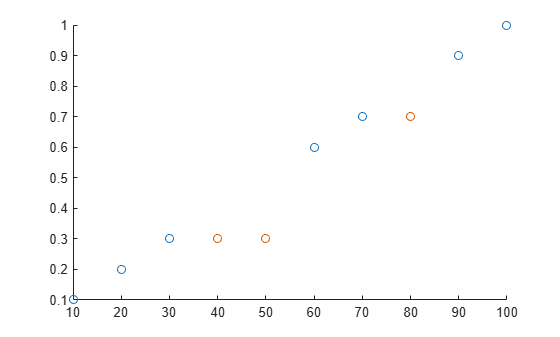```function y = forwardfill(xs,ts,tq,n) % Fill n values in the missing gap using the previous nonmissing value y = NaN(1,numel(tq)); y(1:min(numel(tq),n)) = xs; end```

Create a matrix with missing entries and fill across the columns (second dimension) one row at a time using linear interpolation. For each row, fill leading and trailing missing values with the nearest non-missing value in that row.

```A = [NaN NaN 5 3 NaN 5 7 NaN 9 NaN; 8 9 NaN 1 4 5 NaN 5 NaN 5; NaN 4 9 8 7 2 4 1 1 NaN]```
```A = 3×10 NaN NaN 5 3 NaN 5 7 NaN 9 NaN 8 9 NaN 1 4 5 NaN 5 NaN 5 NaN 4 9 8 7 2 4 1 1 NaN ```
`F = fillmissing(A,'linear',2,'EndValues','nearest')`
```F = 3×10 5 5 5 3 4 5 7 8 9 9 8 9 5 1 4 5 5 5 5 5 4 4 9 8 7 2 4 1 1 1 ```

Fill missing values for table variables with different data types.

Create a table whose variables include `categorical`, `double`, and `char` data types.

```A = table(categorical({'Sunny';'Cloudy';''}),[66;NaN;54],{'';'N';'Y'},[37;39;NaN],... 'VariableNames',{'Description' 'Temperature' 'Rain' 'Humidity'})```
```A=3×4 table Description Temperature Rain Humidity ___________ ___________ __________ ________ Sunny 66 {0x0 char} 37 Cloudy NaN {'N' } 39 <undefined> 54 {'Y' } NaN ```

Replace all missing entries with the value from the previous entry. Since there is no previous element in the `Rain` variable, the missing character vector is not replaced.

`F = fillmissing(A,'previous')`
```F=3×4 table Description Temperature Rain Humidity ___________ ___________ __________ ________ Sunny 66 {0x0 char} 37 Cloudy 66 {'N' } 39 Cloudy 54 {'Y' } 39 ```

Replace the `NaN` values from the `Temperature` and `Humidity` variables in `A` with 0.

`F = fillmissing(A,'constant',0,'DataVariables',{'Temperature','Humidity'})`
```F=3×4 table Description Temperature Rain Humidity ___________ ___________ __________ ________ Sunny 66 {0x0 char} 37 Cloudy 0 {'N' } 39 <undefined> 54 {'Y' } 0 ```

Alternatively, use the `isnumeric` function to identify the numeric variables to operate on.

`F = fillmissing(A,'constant',0,'DataVariables',@isnumeric)`
```F=3×4 table Description Temperature Rain Humidity ___________ ___________ __________ ________ Sunny 66 {0x0 char} 37 Cloudy 0 {'N' } 39 <undefined> 54 {'Y' } 0 ```

Now fill the missing values in `A` with a specified constant for each table variable, which are contained in a cell array.

`F = fillmissing(A,'constant',{categorical({'None'}),1000,'Unknown',1000})`
```F=3×4 table Description Temperature Rain Humidity ___________ ___________ ___________ ________ Sunny 66 {'Unknown'} 37 Cloudy 1000 {'N' } 39 None 54 {'Y' } 1000 ```

Create a time vector `t` in seconds and a corresponding vector of data `A` that contains `NaN` values.

```t = seconds([2 4 8 17 98 134 256 311 1001]); A = [1 3 23 NaN NaN NaN 100 NaN 233];```

Fill only missing values in `A` that correspond to a maximum gap size of 250 seconds. Since the second gap is larger than 250 seconds, the `NaN` value is not filled.

`F = fillmissing(A,'linear','SamplePoints',t,'MaxGap',seconds(250))`
```F = 1×9 1.0000 3.0000 23.0000 25.7944 50.9435 62.1210 100.0000 NaN 233.0000 ```

## Input Arguments

collapse all

Input data, specified as a vector, matrix, multidimensional array, table, or timetable.

When the input argument is a cell array, it must be a cell array of character vectors. If `A` is a timetable, then only table values are filled. If the associated vector of row times contains a `NaT` or `NaN` value, then `fillmissing` produces an error. Row times must be unique and listed in ascending order.

Data Types: `double` | `single` | `int8` | `int16` | `int32` | `int64` | `uint8` | `uint16` | `uint32` | `uint64` | `logical` | `char` | `string` | `cell` | `table` | `timetable` | `categorical` | `datetime` | `duration` | `calendarDuration`

Fill constant, specified as a scalar, vector, or cell array.

`v` can be a vector when `A` is a matrix or multidimensional array, indicating a different fill value for each operating dimension. The length of `v` must match the length of the operating dimension.

`v` can be a cell array of fill values when `A` is a table or timetable, indicating a different fill value for each variable. The number of elements in the cell array must match the number of variables in the table.

Data Types: `double` | `single` | `int8` | `int16` | `int32` | `int64` | `uint8` | `uint16` | `uint32` | `uint64` | `logical` | `char` | `cell` | `categorical` | `datetime` | `duration`

Fill method, specified as one of the following:

MethodDescription
`'previous'`previous non-missing value
`'next'`next non-missing value
`'nearest'`nearest non-missing value
`'linear'`linear interpolation of neighboring, non-missing values (numeric, `duration`, and `datetime` data types only)
`'spline'`piecewise cubic spline interpolation (numeric, `duration`, and `datetime` data types only)
`'pchip'`shape-preserving piecewise cubic spline interpolation (numeric, `duration`, and `datetime` data types only)
`'makima'`modified Akima cubic Hermite interpolation (numeric, `duration`, and `datetime` data types only)

Moving method to fill missing data, specified as one of the following:

MethodDescription
`'movmean'`Moving average over a window of length `window` (numeric data types only)
`'movmedian'`Moving median over a window of length `window` (numeric data types only)

Example: `@(xs,ts,tq) myfun(xs,ts,tq)`

Custom fill method, specified as a function handle. Valid function handles must include the following three input arguments:

Input ArgumentDescription
`xs`Vector containing data values used for filling. The length of `xs` must match the length of the specified window.
`ts`Vector containing locations of the values used for filling. The length of `ts` must match the length of the specified window. `ts` is a subset of the sample points vector.
`tq`Vector containing locations of the missing values. `tq` is a subset of the sample points vector.

The function must return either a scalar or a vector with the same length as `tq`.

Window length for moving methods, specified as a positive integer scalar, a two-element vector of positive integers, a positive duration scalar, or a two-element vector of positive durations. The window is defined relative to the sample points.

When `window` is a positive integer scalar, then the window is centered about the current element and contains `window-1` neighboring elements. If `window` is even, then the window is centered about the current and previous elements. If `window` is a two-element vector of positive integers `[b f]`, then the window contains the current element, `b` elements backward, and `f` elements forward.

When `A` is a timetable or `'SamplePoints'` is specified as a `datetime` or `duration` vector, `window` must be of type `duration`.

Data Types: `double` | `single` | `int8` | `int16` | `int32` | `int64` | `uint8` | `uint16` | `uint32` | `uint64` | `duration`

Gap window length for custom fill functions, specified as a positive integer scalar, a two-element vector of positive integers, a positive duration scalar, or a two-element vector of positive durations. The gap window is defined relative to the sample points.

When specifying a function handle `fillfun` for the fill method, the value of `gapwindow` represents a fixed window length that surrounds each gap of missing values in the input data. The fill value is then computed by `fillfun` using the values in that window. For example, for default sample points `t = 1:10` and data ```A = [10 20 NaN NaN 50 60 70 NaN 90 100]```, a window length `gapwindow = 3` specifies the first window as `[20 NaN NaN 50]` for which `fillfun` operates on to compute the fill value. The second gap window for which `fillfun` operates on is `[70 NaN 90]`.

When `A` is a timetable or `'SamplePoints'` is specified as a `datetime` or `duration` vector, `window` must be of type `duration`.

Data Types: `double` | `single` | `int8` | `int16` | `int32` | `int64` | `uint8` | `uint16` | `uint32` | `uint64` | `duration`

Dimension to operate along, specified as a positive integer scalar. If no value is specified, then the default is the first array dimension whose size does not equal 1.

When `A` is a table or timetable, `dim` is not supported. `fillmissing` operates along each table or timetable variable separately.

Consider a two-dimensional input array, `A`.

• If `dim=1`, then `fillmissing` fills `A` column by column.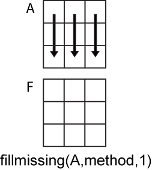• If `dim=2`, then `fillmissing` fills `A` row by row.Data Types: `double` | `single` | `int8` | `int16` | `int32` | `int64` | `uint8` | `uint16` | `uint32` | `uint64`

### Name-Value Arguments

Specify optional comma-separated pairs of `Name,Value` arguments. `Name` is the argument name and `Value` is the corresponding value. `Name` must appear inside quotes. You can specify several name and value pair arguments in any order as `Name1,Value1,...,NameN,ValueN`.

Example: `fillmissing(A,'DataVariables',{'Temperature','Altitude'})` fills only the columns corresponding to the `Temperature` and `Altitude` variables of an input table
Data Options

collapse all

Sample points, specified as the comma-separated pair consisting of `'SamplePoints'` and either a vector of sample point values or one of the options in the following table when the input data is a table. The sample points represent the x-axis locations of the data, and must be sorted and contain unique elements. Sample points do not need to be uniformly sampled. The vector `[1 2 3 ...]` is the default.

When the input data is a table, you can specify the sample points as a table variable using one of the following options.

Option for Table InputDescriptionExamples
Variable name

A character vector or scalar string specifying a single table variable name

`'Var1'`

`"Var1"`

Scalar variable index

A scalar table variable index

`3`

Logical vector

A logical vector whose elements each correspond to a table variable, where `true` specifies the corresponding variable as the sample points, and all other elements are `false`

`[true false false]`

Function handle

A function handle that takes a table variable as input and returns a logical scalar, which must be `true` for only one table variable

`@isnumeric`

`vartype` subscript

A table subscript generated by the `vartype` function that returns a subscript for only one variable

`vartype('numeric')`

Note

This name-value pair is not supported when the input data is a `timetable`. Timetables always use the vector of row times as the sample points. To use different sample points, you must edit the timetable so that the row times contain the desired sample points.

Moving windows are defined relative to the sample points. For example, if `t` is a vector of times corresponding to the input data, then `fillmissing(rand(1,10),'movmean',3,'SamplePoints',t)` has a window that represents the time interval between `t(i)-1.5` and `t(i)+1.5`.

When the sample points vector has data type `datetime` or `duration`, then the moving window length must have type `duration`.

Example: ```fillmissing([1 NaN 3 4],'linear','SamplePoints',[1 2.5 3 4])```

Example: `fillmissing(T,'linear','SamplePoints',"Var1")`

Data Types: `single` | `double` | `datetime` | `duration`

Table variables to operate on, specified as the comma-separated pair consisting of `'DataVariables'` and one of the options in this table. The `'DataVariables'` value indicates which variables of the input table to fill. Other variables in the table not specified by `'DataVariables'` pass through to the output without being operated on.

OptionDescriptionExamples
Variable name

A character vector or scalar string specifying a single table variable name

`'Var1'`

`"Var1"`

Vector of variable names

A cell array of character vectors or string array where each element is a table variable name

`{'Var1' 'Var2'}`

`["Var1" "Var2"]`

Scalar or vector of variable indices

A scalar or vector of table variable indices

`1`

`[1 3 5]`

Logical vector

A logical vector whose elements each correspond to a table variable, where `true` includes the corresponding variable and `false` excludes it

`[true false true]`

Function handle

A function handle that takes a table variable as input and returns a logical scalar

`@isnumeric`

`vartype` subscript

A table subscript generated by the `vartype` function

`vartype('numeric')`

Example: ```fillmissing(T,'linear','DataVariables',["Var1" "Var2" "Var4"])```

Missing Value Options

collapse all

Method for handling endpoints, specified as the comma-separated pair consisting of `'EndValues'` and one of `'extrap'`, `'previous'`, `'next'`, `'nearest'`, `'none'`, or a constant scalar value. The endpoint fill method handles leading and trailing missing values based on the following definitions:

MethodDescription
`'extrap'`same as `method`
`'previous'`previous non-missing value
`'next'`next non-missing value
`'nearest'`nearest non-missing value
`'none'`no fill value
scalarconstant value (numeric, `duration`, and `datetime` data types only)

Data Types: `double` | `single` | `int8` | `int16` | `int32` | `int64` | `uint8` | `uint16` | `uint32` | `uint64` | `logical` | `datetime` | `duration`

Known missing indicator, specified as the comma-separated pair consisting of `'MissingLocations'` and a logical vector, matrix, or multidimensional array of the same size as `A`. The indicator elements can be `true` to indicate a missing value in the corresponding location of `A` or `false` otherwise.

Data Types: `logical`

Maximum gap size to fill, specified as a numeric scalar, `duration` scalar, or `calendarDuration` scalar. Gaps are clusters of consecutive missing values whose size is the distance between the non-missing values surrounding the gap. The gap size is computed relative to the sample points. Gaps smaller than or equal to the max gap size are filled, and gaps larger than the gap size are not.

For example, consider the vector `y = [25 NaN NaN 100]` using the default sample points `[1 2 3 4]`. The gap size in the vector is computed from the sample points as `4 - 1 = 3`, so a `MaxGap` value of `2` leaves the missing values unaltered, while a `MaxGap` value of `3` fills in the missing values.

For missing values at the beginning or end of the data:

• A single missing value at the end of the input data has a gap size of 0 and is always filled.

• Clusters of missing values occurring at the beginning or end of the input data are not completely surrounded by non-missing values, so the gap size is computed using the nearest existing sample points. For the default sample points `1:N`, this produces a gap size that is 1 smaller than if the same cluster occurred in the middle of the data.

## Output Arguments

collapse all

Filled data, returned as a vector, matrix, multidimensional array, table, or timetable. `F` is the same size as `A`.

Data Types: `double` | `single` | `int8` | `int16` | `int32` | `int64` | `uint8` | `uint16` | `uint32` | `uint64` | `logical` | `char` | `string` | `cell` | `table` | `timetable` | `categorical` | `datetime` | `duration` | `calendarDuration`

Filled data indicator, returned as a vector, matrix, or multidimensional array. `TF` is a logical array where 1 (`true`) corresponds to entries in `F` that were filled and 0 (`false`) corresponds to unchanged entries. `TF` is the same size as `A` and `F`.

Data Types: `logical`